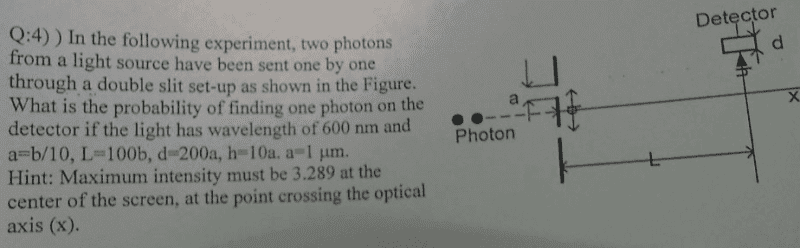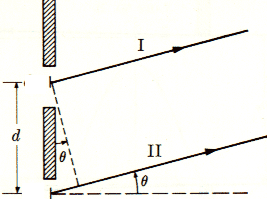# Double slit and Diffraction

grkm

## Homework Statement## Homework Equationsslit width = a , slit seperation = b = d (at photo),
tanQ=h/L m.λ=b.sinQ
λ.b=sinQ
B=π.d.sinQ/λ
α=π.a.sinQ/λ
Iq=Imx(cosB)^2 x (sinα/α)^2//

## The Attempt at a Solution

My first move was to find intensity but I have no idea about the probability of finding one photon on the detector since It is not a point and It has own length.Should I think the detector as a point ? Location can be relevant with fringes but I'm not sure.

#### Attachments

Homework Helper
Gold Member
Finding the intensity is a good first move. Next, think about how the intensity function I(θ) is related to the probability of a photon ending up in some angular interval between θ1 and θ2 after passing through the slits.

grkm
Can I say |I(θ)|^2~P ? and thanks.

Homework Helper
Gold Member
You wouldn't square the intensity. (The intensity, I(θ), already contains the square of the electric field.) When thinking of the probability of where a photon will go, you need to include some interval of angles. For example, if you consider an infinitesimal interval from θ to θ + dθ, then a photon will end up in that interval with a probability that's proportional to I(θ)dθ. For a finite interval from θ1 to θ2, how do you think you would calculate the probability that a photon ends up between θ1 to θ2?

grkm
so interval can be taken from h to h+d ? im not sure but at Imax 's probability is 1/2 im trying to find on detector.

Homework Helper
Gold Member
so interval can be taken from h to h+d ?
Well, you'll need to find the angles θ1 and θ2 that correspond to the distances h and h+d.
im not sure but at Imax 's probability is 1/2 im trying to find on detector.
Sorry, I don't understand this comment.